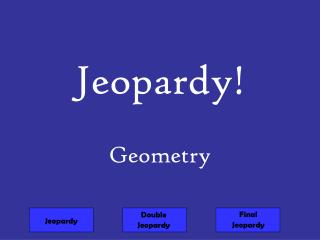DownloadDownload PresentationJeopardy!

# Jeopardy!

Download Presentation## Jeopardy!

- - - - - - - - - - - - - - - - - - - - - - - - - - - E N D - - - - - - - - - - - - - - - - - - - - - - - - - - -
##### Presentation Transcript

1. Jeopardy! Geometry Final Jeopardy Double Jeopardy Jeopardy

2. Jeopardy Double Jeopardy

3. Two lines that intersect at right angles. Answer 10 points/lines

4. Answer • What is perpendicular? 10 points/lines

5. To cross through the same point. Answer 20 points/lines

6. Answer • What is intersect? 20 points/lines

7. A part of a line that has one endpoint and extends forever in the other direction. Answer 30 points/lines

8. Answer • What is a ray? 30 points/lines

9. A flat surface with no thickness that extends without end in all directions on the surface. Answer 40 points/lines

10. Answer • What is a plane? 40 points/lines

11. Daily Double How much would you like to wager?

12. Lines that are not parallel and do not intersect; the lines lie on different planes. Answer

13. Answer • What are skew lines?

14. Which letter is at point (30,40)? Answer 10 points

15. Answer • What is d? 10 points

16. Which coordinate points are outside of the trapezoid? Answer 20 points

17. Answer • What is (5,7)? 20 points

18. Which letter is at position (14,9)? Answer 30 points

19. Answer • What is d ? 30 points

22. What coordinates lie inside the above figure? (7,2) (0,0) (6,4) (5,7) Answer 50 points

23. Answer • What is (6,4)? 50 points

24. 10 sided figure Answer 10 points/polygons

25. Answer • What is a decagon? 10 points/polygons

26. The polygon shown below is called a ____________. Answer 20 points/polygon

27. Answer • What is a pentagon? 20 points/polygons

28. A polygon with six sides. Answer 30 points/polygons

29. Answer • What is a hexagon? 30 points/polygons

31. Answer • What is a polygon? 40 points/polygons

32. A polygon with four sides. Answer 50 points/polygons

34. This angle is ____________. Answer 10 points/angles

35. Answer • What is obtuse? 10 points/angles

36. In the diagram above, angle 3 is a(n) ______ angle. Answer 20 points/angles

37. Answer • What is right angle? 20 points/angles

38. Which angle measure is acute? 91° 89° 110° 90° Answer 30 points/angles

39. Answer • What is B 89° ? 30 points/angles

40. Which angle measure is obtuse? 91° 89° 73° 90° Answer 40 points/angles

41. Answer • What is A 91°? 40 points/angles

42. Which of the angles above is a straight angle? Answer 50 points/angles

43. Answer • What is angle MNO? 50 points/angles

44. Pentagon P is regular. One of its sides has a length of 4 m. What is the perimeter of Pentagon P? Answer 10 points/perimeter

45. Answer • What is 20m? • 4+4+4+4+4=20m • Or 4x5=20m 10 points/perimeter

46. 5 in. 9 in. 6 in. 14 in. What is the perimeter of the triangle? Answer 20 points/perimeter

47. Answer • What is 29 units? • 6+9+14= 29 20 points/perimeter

48. 3 in x= 7 in 5 in 2 in y= Find the missing sides & perimeter. Answer 30 points/perimeter

49. Answer • X= 5-2 = 3 in. • Y= 3 + 7 = 10 in. • P= 3+3+7+2+10+5= 30 in. 30 points/perimeter

50. Hexagon H is regular. One of its sides has a length of 7 ft. What is the perimeter of Hexagon H? Answer 40 points/perimeter